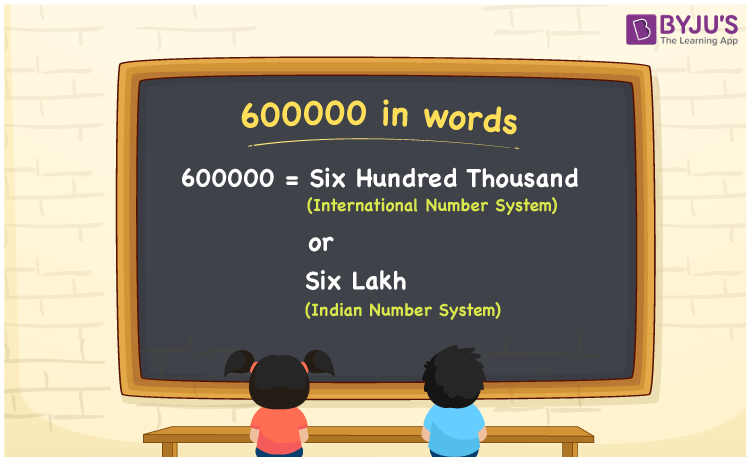# 600000 in Words

The number 600000 in words is six hundred thousand or six lakhs. To convert the number 600000 to words, place values such as ones, tens, hundreds, etc., plays a major role. Hence, we can say that the place value table helps a lot while writing the number 600000 in English words. The number of 600000 can be written using both the Indian Number System and the International Number System. In this article, we are going to learn the spelling of 600000 in detail.

 600000 in Words (International Number System): Six Hundred Thousand 600000 in Words (Indian Number System): Six Lakhs Six Hundred Thousand in Numerical Form: 600000

## 600000 in English Words## How to Write 600000 in Words?

Below is the place value chart for the number 600000. To write the number name of 600000, determine the place value and start converting the number to words.

 Lakhs Ten-thousands Thousands Hundreds Tens Ones 6 0 0 0 0 0

Using the place value chart, the number 600000 is expanded as follows:

= 6 × Lakh + 0 × Ten thousand + 0 × Thousand + 0 × Hundred + 0 × Ten + 0 × One

= 6 × 100000 + 0 × 10000 + 0 × 1000 + 0 × 100 + 0 × 10 + 0 × 1

= 600000

= six hundred thousand

Hence, 600000 in words is six hundred thousand or six lakhs.

The number 600000 is a natural number that appears before 600001 but after 599999.

600000 in words – Six Hundred Thousand or Six Lakhs

Is 600000 an odd number? – No

Is 600000 an even number? – Yes

Is 600000 a perfect square number? – No

Is 600000 a perfect cube number? – No

Is 600000 a prime number? – No

Is 600000 a composite number? – Yes

## Frequently Asked Questions on 600000 in Words

Q1

### How to write 600000 in words as per the International Number System?

According to the International Number System, 600000 in words is six hundred thousand.

Q2

### How to write 600000 in words as per the Indian Number System?

According to the Indian Number System, 600000 in words is six lakhs.

Q3

### How to write six hundred thousand in numbers?

Six hundred thousand in numbers is 600000.